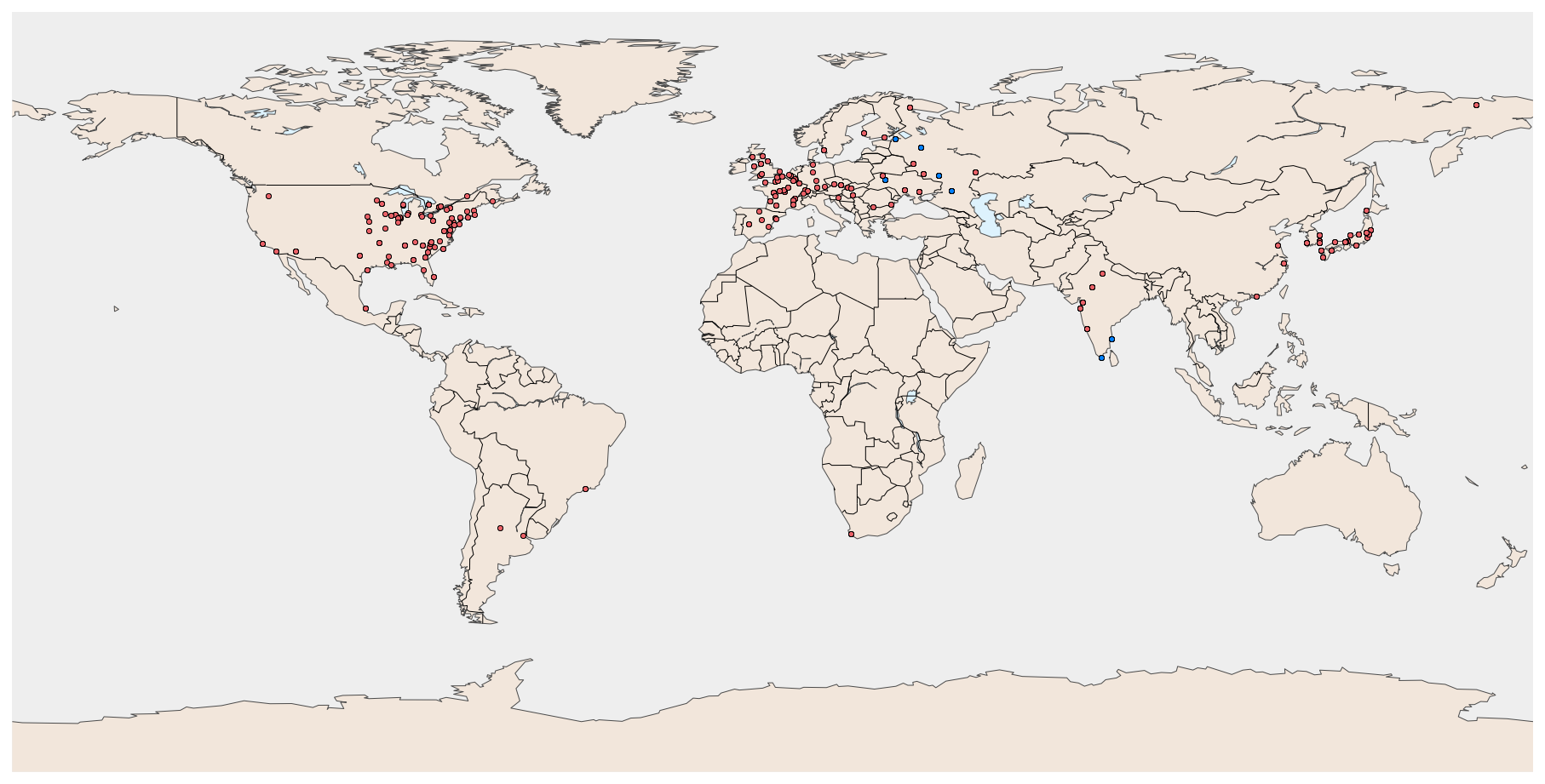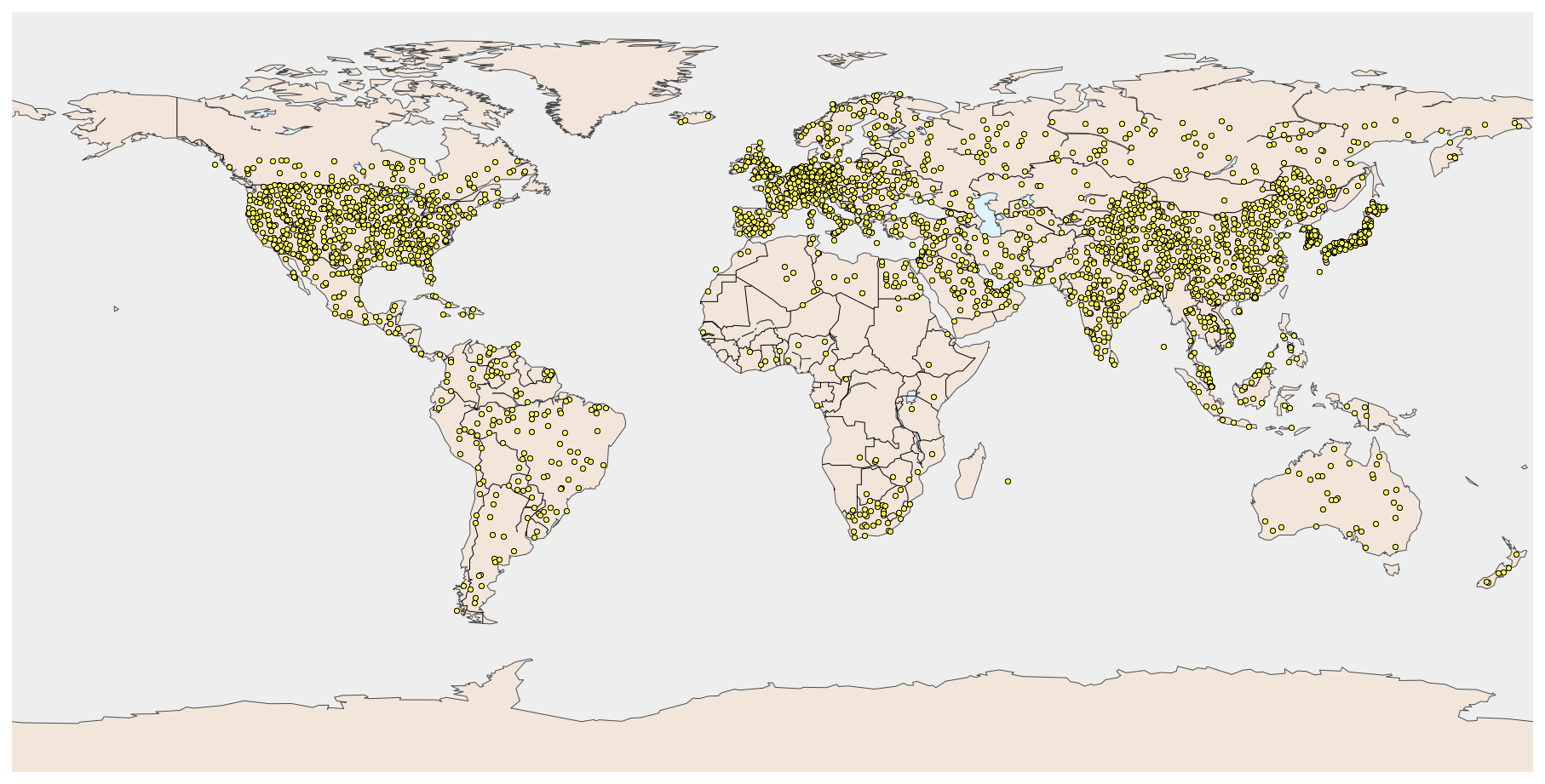13 Apr 2014 #python

# Mapping the world's nuclear power plants

This post demonstrates the use of Python to map the world's nuclear power plants. First, we procure data on plants currently operational or under construction. Then, we make some back of the envelope calculations on how many plants would power the world's current electricity demand.

For this latter part, we use GeoPandas, a very interesting development for Python-based geospatial analysis, building on modules such as Fiona and Shapely to add geospatial functionality on top of one of the workhorses of scientific Python, pandas. From the GeoPandas website:

"The goal of GeoPandas is to make working with geospatial data in python easier. It combines the capabilities of pandas and shapely, providing geospatial operations in pandas and a high-level interface to multiple geometries to shapely. GeoPandas enables you to easily do operations in python that would otherwise require a spatial database such as PostGIS."

See here and here for some more detail on some of the geospatial libraries used and how to install them.

Let's get going! The remainder of this post is based on an IPython notebook available as a GitHub Gist.

First, we set up pylab for inline plotting, and then import quite a few modules:

• pandas and geopandas to provide our basic tabular data structure
• matplotlib and Basemap for plotting
• sparql to execute SPARQL queries
• shapely for its geospatial geometry objects
• requests to make HTTP requests
In :
```%pylab inline
```
```Populating the interactive namespace from numpy and matplotlib
```
In :
```import random
import json

import matplotlib.pyplot as plt
from mpl_toolkits.basemap import Basemap
import pandas as pd
import geopandas as gp
import sparql
from shapely import geometry
import requests
```

## Get power plant data¶

Enipedia is a wiki with freely available data on energy, run by TU Delft. The database contains information on power plants around the world aggregated from various sources, and is accessible via SPARQL, a web-based database query language, so it is ideal for our purposes.

Using Enipedia’s SPARQL endpoint, the SPARQL query below selects plants from the general Enipedia database and matches them up with plants from IAEA (Imnternational Atomic Energy Agency) data contained in Enipedia to get their operational status.

In :
```s = sparql.Service('http://enipedia.tudelft.nl/wiki/Special:SparqlExtension')
```
In :
```statement = """
PREFIX prop: <http://enipedia.tudelft.nl/wiki/Property:>
PREFIX a: <http://enipedia.tudelft.nl/wiki/>
PREFIX rdf: <http://www.w3.org/1999/02/22-rdf-syntax-ns#>
PREFIX iaea: <http://enipedia.tudelft.nl/data/IAEA/>
PREFIX rdfs: <http://www.w3.org/2000/01/rdf-schema#>

SELECT ?Name ?Plant ?Lat ?Lon ?Status WHERE {
GRAPH <http://enipedia.tudelft.nl/wiki/> {
?Plant prop:IAEA_Name ?IAEAName .
?Plant prop:Latitude ?Lat .
?Plant prop:Longitude ?Lon .
}
GRAPH <http://enipedia.tudelft.nl/data/IAEA> {
?x rdfs:label ?Name .
FILTER(?Name = ?IAEAName) .
?x iaea:status ?Status .
}
}
"""
```

For easy processing, the query result is directly turned into a pandas DataFrame.

In :
```result = s.query(statement)
df = pd.DataFrame(result.fetchall(), columns=result.variables)
```

We then convert the SPARQL literals to regular Python strings, and the Lat and Lon columns to floats:

In :
```for col in ('Name', 'Plant', 'Status'):
df[col] = df[col].map(lambda x: str(x))

for col in ('Lat', 'Lon'):
df[col] = df[col].map(lambda x: float(str(x)))
```

Let’s see what types of Status the data contain:

In :
```df.Status.unique()
```
Out:
```array(['Operational', 'Long-term Shutdown', 'Permanent Shutdown',
'Under Construction'], dtype=object)```

We only want to keep plants that are operating or under construction, so we select all data where Status does not contain `'Shutdown'` (by using the `~` operator to negate `str.contains`):

In :
```df = df[~df.Status.str.contains('Shutdown')]
```
In :
```df.info()
```
```<class 'pandas.core.frame.DataFrame'>
Int64Index: 418 entries, 0 to 463
Data columns (total 5 columns):
Name      418 non-null object
Plant     418 non-null object
Lat       418 non-null float64
Lon       418 non-null float64
Status    418 non-null object
dtypes: float64(2), object(3)```

## Plotting the plants¶

We are left with about 400 nuclear plants. The next step is to set up the map.

In :
```m = Basemap(projection='cyl', ellps='WGS84',
llcrnrlon=-180, llcrnrlat=-90, urcrnrlon=180, urcrnrlat=90,
resolution='c', suppress_ticks=True)
```
In :
```fig = plt.figure(figsize=(16, 16))
m.drawmapboundary(fill_color=None, linewidth=0)
m.drawcoastlines(color='#4C4C4C', linewidth=0.5)
m.drawcountries()
m.fillcontinents(color='#F2E6DB',lake_color='#DDF2FD')
for index, site in df.iterrows():
y, x = m(site.Lat, site.Lon)  # Translate lat/lon to basemap coordinates
if site.Status == 'Operational':
color = '#EA6167'
else:
color = '#0080FF'
m.plot(x, y, marker='o', markersize=3.0, alpha=1.0, color=color)
```This is not completely accurate — some plants in planning or under construction are missing, e.g. in China, but it gives a fairly good picture of where currently existing reactors are (note that there is only one in all of Africa, near Cape Town).

## Plants to power the world¶

Let’s look at the hypothetical question of how many nuclear power plants could power the world. We’ll ignore a lot of practical issues and use this only as a very simple thought experiment.

We need three types of data:

• Country outlines: available from a Natural Earth
• Per-country electricity production data, from the World Bank
• The capacity of a typical nuclear power plant, which we simply assume

We will use a simple approach to randomly place nuclear power plants in each country to match that country’s electricity demand (of course, this means plants may end up in the center of large cities or in the middle of deserts, far from access to cooling water — but we will disregard these minor details).

First, we load Natural Earth countries into a GeoDataFrame with geopandas. A GeoDataFrame is a geospatially-aware pandas DataFrame, which makes it easier to deal with spatial data and perform simple GIS operations with the geometries it contains.

This assumes that you have downloaded the ne_10m_admin_0_countries dataset from Natural Earth into a folder called `ne_10m_admin_0_countries`.

In :
```geodf = gp.read_file('ne_10m_admin_0_countries/ne_10m_admin_0_countries.shp')
```
In :
```# Fix Taiwan and Norway 2-letter country codes

geodf.at[taiwan_index, 'WB_A2'] = 'TW'
geodf.at[norway_index, 'WB_A2'] = 'NO'
```
In :
```geodf.index = geodf['WB_A2']  # Set two-letter country code as index
```

The World Bank has data on global electricity production in kWh, which we can access via their public API, choosing the year 2010:

In :
```wb_url = ('http://api.worldbank.org/countries/indicators/'
'EG.ELC.PROD.KH?per_page=1000&date=2010:2010&format=json')

r = requests.get(wb_url)
elec = pd.DataFrame(wb_data)  # Create a pandas DataFrame from dictionary
```

Some cleanup is needed. First, the ‘country’ column contains dictionaries with multiple keys, but we only want to keep the name of the country. Second, we drop all the unnecessary columns, and reindex the DataFrame with country names.

In :
```elec['code'] = elec.country.map(lambda x: x['id'])
elec['country'] = elec.country.map(lambda x: x['value'])
elec = elec.drop(['decimal', 'date', 'indicator'], axis=1)
elec = elec.set_index('code')
```
In :
```elec.info()
```
```<class 'pandas.core.frame.DataFrame'>
Index: 252 entries, 1A to ZW
Data columns (total 2 columns):
country    252 non-null object
value      163 non-null object
dtypes: object(2)```

Only 163 rows out of 252 have a non-null value, so there is quite a bit of missing data, but we’ll ignore that and simply keep only non-null values.

In :
```elec = elec.dropna()
```

Finally, we assume that the nuclear plants have a capacity of 1 GW and a capacity factor of 90% (i.e., they are up and running for 90% of the time). The power output of a plant in kWh per year is therefore:

In :
```production_per_plant = 1000000 * 8760 * 0.9
```

For each country, we divide the total electricity production by the electricity produced from our typical nuclear plant to find the number of plants needed to power that country:

In :
```elec['Nuclear_Plants'] = np.ceil(elec.value.astype(float) / production_per_plant)
```

Now we match up the countries from `elec` with those in `geodf`:

In :
```for country in geodf.index:
try:
geodf.at[country, 'points'] = elec.at[country, 'Nuclear_Plants']
except KeyError:
geodf.at[country, 'points'] = 0
```

Finally, we randomly place nuclear plants in each country. To do so, we iterate over each row in the GeoDataFrame. For each row, we keep generating random points, keeping those that are contained by the row’s geometry (the outline of the current country), until we’ve reached the number of plants for the current country.

In the United States, Canada, Russia and Norway, we manually exclude some of the northernmost parts (such as Alaska).

In :
```random_points = []
for index, row in geodf.iterrows():
found = 0
while found < row.points:
x_max, y_max, x_min, y_min = row.geometry.bounds
if index == 'US':
y_min = 50.0  # Exclude all but southern coastal Alaska
elif index == 'RU':
y_min = 65.0  # Exclure northern parts
elif index == 'CA':
y_min = 55.0  # Exclude northern parts
elif index == 'NO':
y_min = 73.0  # Exclude Svalbard islands
point = geometry.Point(x_min + (random.random() * (x_max - x_min)),
y_min + (random.random() * (y_max - y_min)))
if row.geometry.contains(point):
found += 1
random_points.append(point)
```
In :
```len(random_points)
```
Out:
`2734`

So, we have about 2700 nuclear power plants to power the world (or at least, the countries we had data for).

In :
```fig = plt.figure(figsize=(16, 16))

m.drawmapboundary(fill_color=None, linewidth=0)
m.drawcoastlines(color='#4C4C4C', linewidth=0.5)
m.drawcountries()
m.fillcontinents(color='#F2E6DB',lake_color='#DDF2FD')

for p in random_points:
m.plot(p.x, p.y, marker='o', markersize=3.0, alpha=1.0, color='#FEF750')
```This post has used a practical example to demonstrate how Python and the IPython notebook can be used to quickly gather, combine and visualize data in an exploratory way.